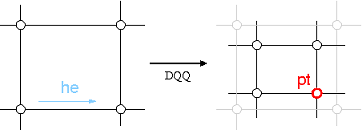CGAL 4.14 - 3D Surface Subdivision Methods
CGAL::DooSabin_mask_3< PolygonMesh, VertexPointMap > Class Template Reference

Definition

template<class PolygonMesh, class VertexPointMap = typename boost::property_map<PolygonMesh, vertex_point_t>::type> class CGAL::DooSabin_mask_3< PolygonMesh, VertexPointMap >

The geometry mask of Doo-Sabin subdivision.

A stencil determines a source neighborhood whose points contribute to the position of a refined point. The geometry mask of a stencil specifies the computation on the nodes of the stencil. DooSabin_mask_3 implements the geometry masks of Doo-Sabin subdivision on models of MutableFaceGraph, such as Polyhedron_3 and Surface_mesh.

Template Parameters
 PolygonMesh must be a model of the concept MutableFaceGraph. VertexPointMap must be a model of WritablePropertyMap with value type Point_3Is Model Of:
CGAL::Subdivision_method_3

Public Types

typedef PolygonMesh MeshPublic Types inherited from CGAL::DQQ_stencil_3< PolygonMesh, VertexPointMap >
typedef PolygonMesh Mesh

typedef boost::property_map< Mesh, vertex_point_t >::type Vertex_pmap

typedef boost::graph_traits< Mesh >::vertex_descriptor vertex_descriptor

typedef boost::graph_traits< Mesh >::halfedge_descriptor halfedge_descriptor

typedef boost::graph_traits< Mesh >::face_descriptor face_descriptor

typedef boost::property_traits< Vertex_pmap >::value_type Point

typedef Kernel_traits< Point >::Kernel Kernel

typedef Kernel::FT FT

typedef Kernel::Vector_3 Vector

Creation

Constructor. More...

Constructor with a custom vertex point property map.

Stencil functions

void corner_node (halfedge_descriptor he, Point &pt)
computes the Doo-Sabin point pt of the vertex pointed by the halfedge he.Public Member Functions inherited from CGAL::DQQ_stencil_3< PolygonMesh, VertexPointMap >
DQQ_stencil_3 (Mesh *pmesh)

DQQ_stencil_3 (Mesh *pmesh, VertexPointMap vpmap)Public Attributes inherited from CGAL::DQQ_stencil_3< PolygonMesh, VertexPointMap >
Mesh * pmesh

Vertex_pmap vpm